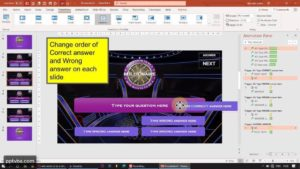# Shuffle Questions Slides & Answer Order Randomly in PPT Quiz Game

In this Interactive PowerPoint Quiz Game Module, we will be shuffling the order of the questions such that all the questions are shown to the students with no repetition. We achieve this by generating random orders and moving slides to a random position within a given range.

To generate the order of those multiple choice answer boxes, we rename those four boxes as “a1, a2, a3, a4”. And then, we make an array and shuffle that to get all the 16 combinations of “1,2,3,4”. Based on the order of the sequence, the answer boxes: “a1, a2, a3, a4” are arranged in slide-show mode.a

### Shuffle Answers, Multiple Attempts Customisable PPT Quiz Template

The student can keep answering the question until their answer is correct.

You can also change the colours to match your theme. Duplicate the slides and just type your own question!

Send Report Card to Google Sheets, Import Questions from Excel.
Make your quiz game in 54 seconds!

## Randomise Answer Order in PowerPoint Question Slides

``````Sub RandomiseAnswerOrder()
ReDim AnswerOrder(3) '0 1 2 3 -> 4 compartments'

For i = 0 To 3
Next i

For i = 3 To 8

Randomize
For N = 0 To 3
J = Int(4 * Rnd) 'random number from 0 to 3

Next N

For j = 0 To 3

ActivePresentation.Slides(i).Shapes("a" & j+1).Top = 218
ActivePresentation.Slides(i).Shapes("a" & j+1).Left = 303
ActivePresentation.Slides(i).Shapes("a" & j+1).Top = 288
ActivePresentation.Slides(i).Shapes("a" & j+1).Left = 303
ActivePresentation.Slides(i).Shapes("a" & j+1).Top = 363
ActivePresentation.Slides(i).Shapes("a" & j+1).Left = 303
ActivePresentation.Slides(i).Shapes("a" & j+1).Top = 432
ActivePresentation.Slides(i).Shapes("a" & j+1).Left = 303
End If

Next j
Next i

End Sub ``````

I put the codes used to randomize the array inside the For i = 3 To 8  Loop: Or else, the answer boxes were shuffled in the same manner across all the slides.

If the order of the answers were: a3, a2, a1 and a4 in your first slide, it would been the same order for all the other questions slides too.

However, by randomizing the array every time for a new slide, we are able to shuffle the answer order in a new different order.

## Randomise Question Slides in PowerPoint Quiz Game

``````Sub ShuffleSlides()
FirstSlide = 3
LastSlide = 8

Randomize
'generate random number between 2 to 7'
RSN = Int((LastSlide - FirstSlide + 1) * Rnd + FirstSlide)

For i = FirstSlide To LastSlide
ActivePresentation.Slides(i).MoveTo (RSN)
Next i
End Sub ``````

## Change Name of Shapes Automatically in PowerPoint

We can change the name of the shape in Microsoft PowerPoint by using the following VBA Macro:

``````Sub ChangeNameOfShape()
For i = 3 To 8
ActivePresentation.Slides(i).Shapes("Rect 5").Name = "a1"
Next i
End Sub ``````

## Find Position of Answer Shapes in PowerPoint Quiz Game

We can find the position of the answer shape by printing the co-ordinates of the shape in our Immediate Window. Toggle that by pressing Ctrl + G in your Visual Basic Applications Window

``````Sub FindPositionOFAnswers()
Debug.Print ActivePresentation.Slides(3).Shapes("a1").Top
Debug.Print ActivePresentation.Slides(3).Shapes("a2").Top
Debug.Print ActivePresentation.Slides(3).Shapes("a3").Top
Debug.Print ActivePresentation.Slides(3).Shapes("a4").Top
End Sub ``````

### Shuffling sequence of 1,2,3,4 by shuffling an Array in an Random Order

Arrays can store multiple values in different compartments. The following is an example of the array which we used to randomise the answer order by shuffling the sequence of “1,2,3,4”.We generate a random compartment number from 0 to 3 and take the value of that compartment and place it in compartment 0. We generate another random compartment number and take its value and place it in compartment 1. Similarly, we do these via a For-Loop:

``For N = 0 To 3 ``

We transfer the values from one compartment to another by storing the value in a temporary variable called temp

``````temp = AnswerOrder(N)

In my above code where I assign the position, i = 3 To 8 refers to slides that contain questions. A loop is made for every value of i, which is: 3, 4, 5, 6, 7, 8.

j = 0 To 3 refers to the compartment numbers (the correct terminology is indexing) of the array. The compartment 0 corresponds to the shape a1, compartment 1 corresponds to the shape a2… and so on!

Thus, we refer to the shape as “a” & j + 1 since a very visible relation is possible:
If j = 0, shape = “a” & j + 1 = “a” & 0 + 1 = “a1” and so on…

Thus, our PowerPoint VBA Macro Code to shuffle the answer orders becomes much more neat. You can also use the similar trick of shuffling an array to randomly shuffle the question slides without literally causing the slides to change their position.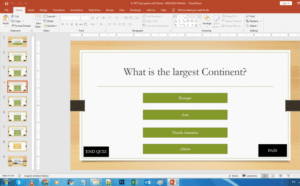## Interactive PowerPoint Quiz Game with Points and Scores

In this PowerPoint Quiz Module, we will be designing our...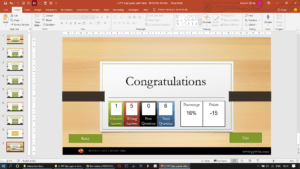## REPORT CARD AND PERCENTAGE – INTERACTIVE POWERPOINT QUIZ GAME

In this PowerPoint Quiz Game, we will calculate the number...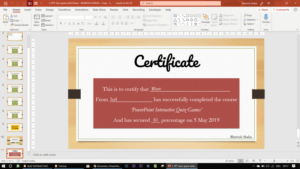## PRINT CERTIFICATE IN PPT QUIZ GAME

Collect student's name via an input field and use VBA...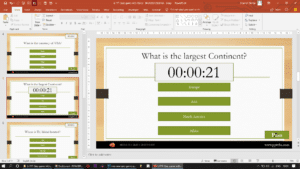## POWERPOINT QUIZ GAME WITH TIMER

As soon as the time limit is up, the student...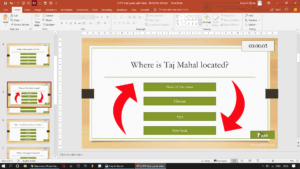## SHUFFLE QUESTIONS SLIDES & ANSWER ORDER RANDOMLY IN PPT QUIZ GAME​

We will be shuffling the order of the questions such...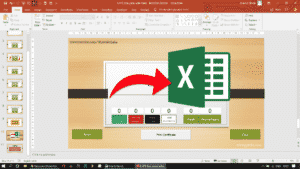## SAVE POWERPOINT QUIZ RESULTS TO EXCEL SHEET​

We will be exporting/sending the results of the PowerPoint Quiz...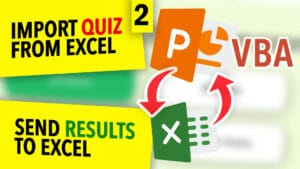## Populate A PowerPoint Quiz From Excel With VBA

Import Questions & Answers of a Microsoft PowerPoint Multiple-Choice Quiz...